Year 8 Interactive Maths - Second Edition

## Number Patterns

A sequence is a pattern of numbers that are formed in accordance with a definite rule.

We can often describe number patterns in more than one way.  To illustrate this, consider the following sequence of numbers {1, 3, 5, 7, 9, …}.

Clearly, the first term of this number pattern is 1; and the terms after the first term are obtained by adding 2 to the previous term.  We can also describe this number pattern as a set of odd numbers.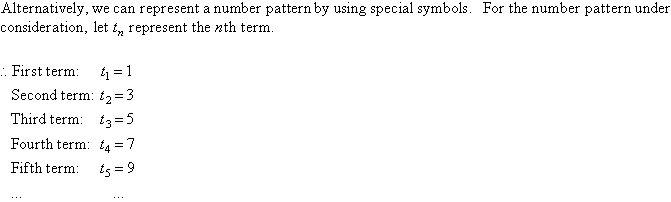By trial and error, we find that: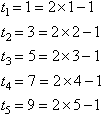By observation, we notice that we can describe this number pattern by the rule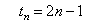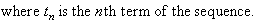### Formula and Tables

A table of values can be generated from the ruleas shown below.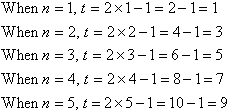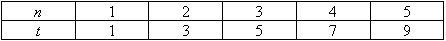## Finding the Algebraic Rule

We use algebra to study rules that describe the behaviour of everyday things.  For example, the behaviour of the height of a ball when it is thrown upward or the amount outstanding for a loan after a number of regular repayments.  By finding a pattern in observed values (i.e. measurements), we are often able to discover a rule that allows us to make accurate predictions.

### Using a Difference Pattern

When we try to discover an algebraic rule for ordered pairs, we can find the difference between two successive values of y.  This allows us to find a rule as illustrated below.

Consider the following table.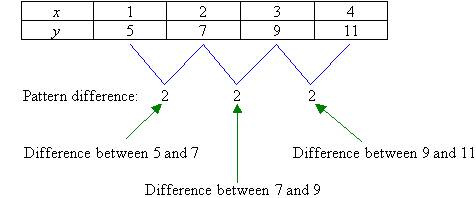We notice that the values of x increase by just one at a time and the difference between the successive values for y is 2.  So, the rule starts off with y = 2x.  Will this give a correct answer from the table?  Let us check.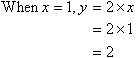The answer is no.  From the table, when x = 1 the value of y should be 5.  How do we change our answer from 2 to 5?  We should add 3.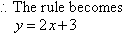Check the rule to see if it is correct: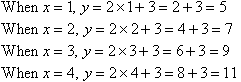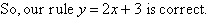#### Example 5

Discover the rule for the following table of values: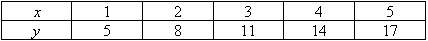##### Solution:

In the given table, the x-values increase by 1 for each ordered pair.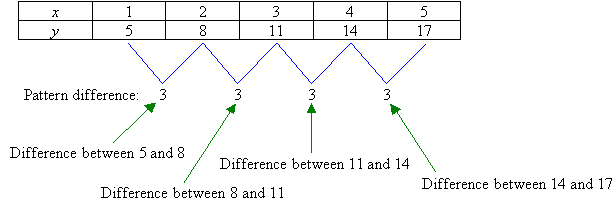Find the difference between the successive values of y.  That is: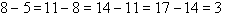The difference between successive values of y is always 3.  So, the rule is of the form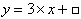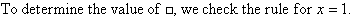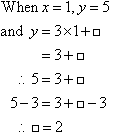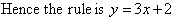###### Check:

Check the rule to see if it is correct: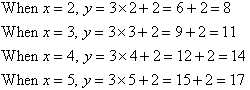So, our rule is correct.

###### Note:

To establish a rule for a number pattern involving ordered pairs of x and y, we can find the difference between every two successive values of y.  If the difference pattern is the same, then the coefficient of x in the algebraic rule (or formula) is the same as the difference pattern.

###### Key Terms

Copyright © 2000-2019 mathsteacher.com Pty Ltd.  All rights reserved.
Australian Business Number 53 056 217 611

Copyright instructions for educational institutions

Please read the Terms and Conditions of Use of this Website and our Privacy and Other Policies.
If you experience difficulties when using this Website, tell us through the feedback form or by phoning the contact telephone number.